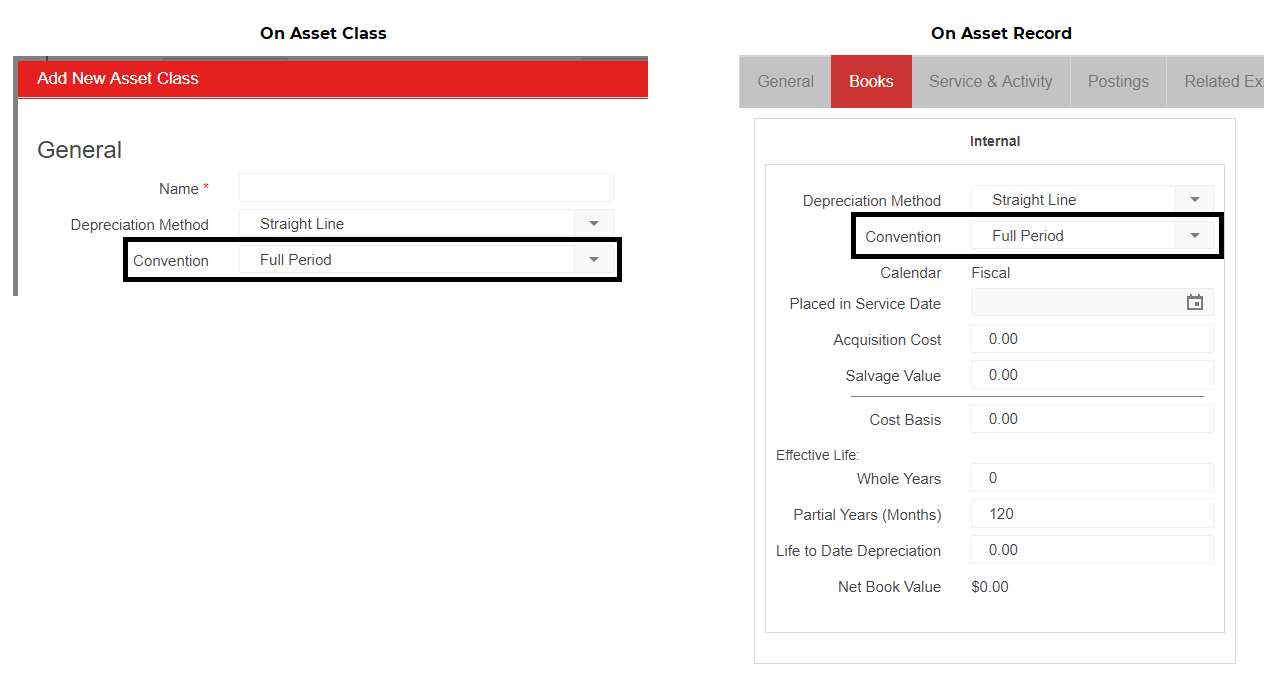The Averaging Convention is selected on each Asset Book and is used to determine the following:

• What is the Depreciation Start Date for this Book?
• How much Depreciation should be included in the First and Last Months of Depreciation?

Below are the descriptions for each Averaging Convention and how each Convention determines the answer for the above questions.  Below each Convention description is an example Start and End date for the Convention type.  All examples assume the following:

1. Fiscal Year Type: Calendar Year
2. Asset Effective Life: 2 Years, 0 Months
3. Placed in Service Date: 1/10/2017

Conventions:

• Full Period = Depreciation start is 1st day of Period that the Asset is 'Placed in Service'
• Depreciation Start = 1/1/2017
• Depreciation End = 12/31/2018
• Mid Period Half = Depreciation start occurs any day in a Period where the Asset is 'Placed in Service' (between days 1 and 30) and receives a Half Depreciation for that Period.  Regular Depreciation occurs the following Period.  The final Period also receives a Half Period of Depreciation
• Depreciation Start = 1/18/2017 (Half Depreciation in this period)
• Depreciation End = 1/17/2019 (Half Depreciation in this period)
• Mid Period Full = Depreciation start depends on which day the Asset is 'Placed in Service'.  For days 1 - 15: the Asset receives a Full Period of Depreciation.  For days 16 - 30: the Assets receives No Depreciation for the Period and will start Depreciation the following Period
• Depreciation Start = 1/18/2017 (Date occurs after Day 16, therefore No Depreciation in this period)
• Depreciation End = 1/17/2019
• Next Period = Depreciation start is 1st day of Period following the Period that the Asset is 'Placed in Service'
• Depreciation Start = 2/1/2017
• Depreciation  End = 1/31/2019